# System of linear equations facts for kids

Kids Encyclopedia Facts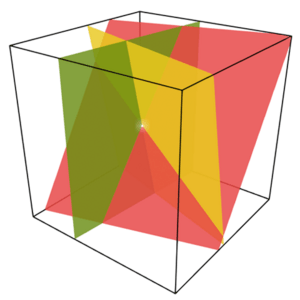A linear system in three variables determines a collection of planes (one plane for each equation). The intersection point is the solution.

In mathematics, a system of linear equations (or linear system) is a collection of linear equations involving the same set of variables.

## Description

Mathematicians show the relationship between different factors in the form of equations. "Linear equations" mean the variable appears only once in each equation without being raised to a power. A "system" of linear equations means that all of the equations are true at the same time. So, the person solving the system of equations is looking for the values of each variable that will make all of the equations true at the same time. If no such values can satisfy all of the equations in the system, then the equations are called "inconsistent."

For example,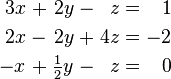\begin{alignat}{7} 3x &&\; + \;&& 2y &&\; - \;&& z &&\; = \;&& 1 & \\ 2x &&\; - \;&& 2y &&\; + \;&& 4z &&\; = \;&& -2 & \\ -x &&\; + \;&& \tfrac{1}{2} y &&\; - \;&& z &&\; = \;&& 0 & \end{alignat}

is a system of three equations in the three variables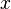$x$,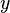$y$,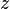$z$. A "solution" to a linear system is an assignment of numbers to the variables such that all the equations are simultaneously satisfied. A solution to the system above is given by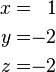\begin{alignat}{2} x & = & 1 \\ y & = & -2 \\ z & = & -2 \end{alignat}

since it makes all three equations valid: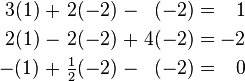\begin{alignat}{7} 3(1) &&\; + \;&& 2(-2) &&\; - \;&& (-2) &&\; = \;&& 1 & \\ 2(1) &&\; - \;&& 2(-2) &&\; + \;&& 4(-2) &&\; = \;&& -2 & \\ -(1) &&\; + \;&& \tfrac{1}{2} (-2) &&\; - \;&& (-2) &&\; = \;&& 0 & \end{alignat}

In mathematics, the theory of linear systems is a branch of linear algebra, a subject which is fundamental to modern mathematics. Computer algorithms for finding the solutions are an important part of numerical linear algebra, and such methods play a prominent role in engineering, physics, chemistry, computer science, and economics. A system of non-linear equations can often be approximated by a linear system (see linearization), a helpful technique when making a mathematical model, computer model, or computer simulation of a relatively complex system. For complex systems, there are many equations and many variables, not just two or three. In many cases, the number of equations and variables in the system are the same. In some cases, there are more variables than equations, and the solution will be a range of different values rather than one exact solution.

## Simple example

The simplest kind of linear system involves two equations and two variables: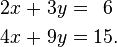\begin{alignat}{5} 2x &&\; + \;&& 3y &&\; = \;&& 6 & \\ 4x &&\; + \;&& 9y &&\; = \;&& 15&. \end{alignat}

One method for solving such a system is as follows. First, solve the top equation for$x$ in terms of$y$: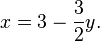$x = 3 - \frac{3}{2}y.$

Now substitute this expression for x into the bottom equation: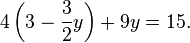$4\left( 3 - \frac{3}{2}y \right) + 9y = 15.$

This results in a single equation involving only the variable$y$. Solving gives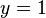$y = 1$, and substituting this back into the equation for$x$ yields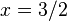$x = 3/2$: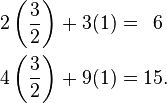\begin{alignat}{5} 2\left(\frac{3}{2}\right) &&\; + \;&& 3(1) &&\; = \;&& 6 & \\ 4\left(\frac{3}{2}\right) &&\; + \;&& 9(1) &&\; = \;&& 15&. \end{alignat}

This method generalizes to systems with additional variables.

## Using Matrices

Very often, all the coefficients are written in the form of a matrix A, which is called a coefficient matrix.$A = \begin{bmatrix} a_{11} & a_{12} & \cdots & a_{1n}\\ a_{21} & a_{22} & \cdots & a_{2n}\\ \vdots & \vdots & \ddots & \vdots\\ a_{m1} & a_{m2} & \cdots & a_{mn} \end{bmatrix}$

In much the same way, the variables can be written in the form of a vector: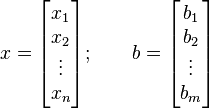$x = \begin{bmatrix} x_1\\ x_2 \\ \vdots \\ x_n\end{bmatrix};\qquad b = \begin{bmatrix} b_1\\ b_2 \\ \vdots \\ b_m\end{bmatrix}$.

This makes it possible to write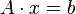$A \cdot x = b$.

Mathematically, the vector defined above is a 1-by-n matrix. The system of equations can then be solved using the multiplication operation defined on matrices. A, x and b are all part of the same algebraic field.

## Solving a system of linear equations

There a three cases when looking for solutions to a system of linear equations:

• There is no solution
• There is exactly one solution
• There are many solutions; the exact number depends on the properties of the field. In many cases there will be an infinite number of solutions.

There are two categories of methods for solving a system of linear equations. Iterative methods use many steps to get a solution, direct methods only need one step:

• An example for a direct method is to solve the system for one variable; this variable can be eliminated and replaced by an expression that only uses other variables, or a number. Doing this for all variables of the equation will lead to a solution of the system if it exists.
• Another method is to transform two equations so that one of the sides of the equations is the same in both cases; it is then possible to write another equation, which replaces the two equations and reduces the number of equations by one.
• Gaussian elimination
• QR decomposition
• Cholesky decomposition
• Cramer's rule

Examples for iterative methods are:

• Relaxation, including the Gauss-Seidel and Jacobi methods
• Multigrid method
• Krylow method

There are examples such as geodesy where there many more measurements than unknowns. Such a system is almost always overdetermined and has no exact solution. Each measurement is usually inaccurate and includes some amount of error. Since the measurements are not exact, it is not possible to obtain an exact solution to the system of linear equations; methods such as Least squares can be used to compute a solution that best fits the overdetermined system. This least squares solution can often be used as a stand-in for an exact solution.

Solving a system of linear equations has a complexity of at most O (n3). At least n2 operations are needed to solve a general system of n linear equations. The best algorithm known to date was developed by Don Coppersmith and Shmuel Winograd and dates from 1990. It has a complexity of n2.376 Unfortunately, it is of little practical use.

## Uses

Using computers to solve systems of linear equations is used every day. For example, it is used in weather forecasting models. Hot dog factories use it to make small changes in the receipe as food ingredient prices change. College cafeterias use it to figure out how much food to cook based on past experience when the cafeteria gives students the choice between multiple entrees.

## Related pagesSystem of linear equations Facts for Kids. Kiddle Encyclopedia.